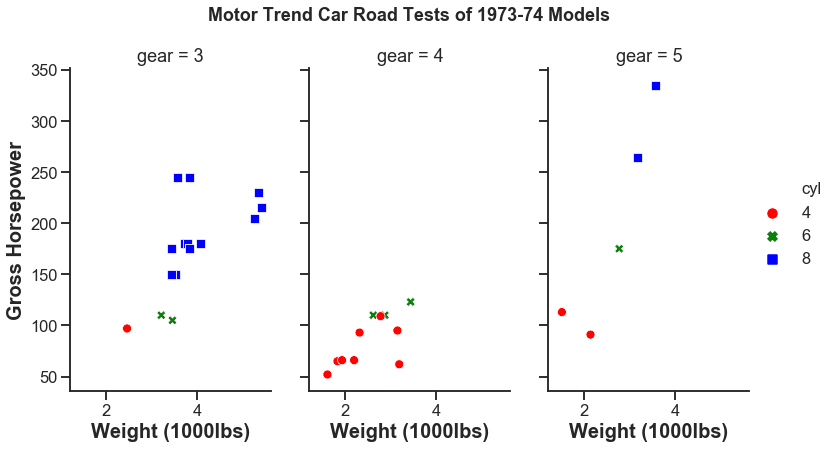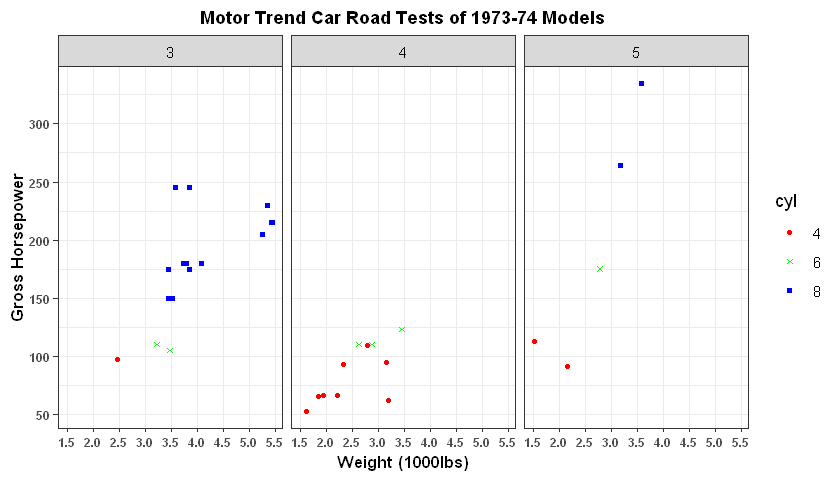#R vs Python for Data Visualization

This article demonstrates creating similar plots in R and Python using two of the most prominent data visualization packages on the market, namely ggplot2 and Seaborn.commentsggplot2 vs seaborn

R and Python have inundated us with the ability to generate complex and attractive statistical graphics in order to gain insights and explore our data. Both are well equipped to handle millions of data points (maybe billions depending on the platform).

### Visualizing Data in Python

Seaborn is built on top of Matplotlib and is a comparatively simpler syntax and structure to Matplotlib.

First we use `import seaborn as sns; sns.set()` to load and set the seaborn theme defaults to the Python session. Matplotlib has to be loaded as well since both libraries are used in tandem.

```    import seaborn as sns; sns.set()
import matplotlib.pyplot as plt
```

1. `sns.set_style()` sets the background theme of the plot. "ticks" is the closest to the plot made in R.
2. `sns.set_context()` will apply predefined formatting to the plot to fit the reason or context the visualization is to be used. `font_scale=1` is used to set the scaele of the font size for all the text in the graph.
3. `plt.figure()`is a command to control different aspects of the matpltlib graph (as stated before seaborn graphs are just Matplotlib plots under the hood).
4. `sns.scatterplot()`is the command used to pass arguments to create the seaborn style scatterplot.
• `x="wt"`maps the weight to the x-axis.
• `y="hp"`maps the horsepower to the y-axis.
• `hue="cyl"`will fill and color the scatterpoints.
• `palette=['red','green','blue']`manually overrides the color palette that is set by `hue`to red, green and blue.
• `data="mtcars"`allows us to use the data in the dataset.
• `style='cyl'`assigns shapes to each cyinder category.
• `legend='brief'`will assign the `hue`&`size`.
5. `sizes=(800,1000)`
6. controls the minimim and maximum size of the scatterpoints on the plot.

7. `plt.title()` gives the plot its main title. If you are an experienced Matplotlib user or used plt.suptitle() before you know the confusion when using the two together. The arguments are self-explanatory.
8. `plt.xlabel()`will format the x-axis label. I use `set_..` to access the class to include the aesthetic properties. This can get cluttered at times but there are many ways to format a seaborn/matplotlib plot. This is useful for after the plot has been created. The plot was already made with `sns.scatterplot` so now we need to override the default formats in this manner.
9. `plt.ylabel()`works in the exact same way just for the y-axis.
```    #sns.set_style("darkgrid")
sns.set_style("ticks")
sns.set_context("talk")
#sns.set_context("notebook", font_scale=1)
plt.figure(figsize=(10,8)) #plt.figure(figsize=(width,height))
sns.scatterplot(x="wt", y="hp", hue='cyl', palette=['red','green','blue'],
data=mtcars, style='cyl', legend='brief', sizes=(800,1000)
)
plt.title('Motor Trend Car Road Tests of 1973-74 Models', fontsize=24, fontweight='bold')
plt.xlabel("Weigt (1000lbs)").set_fontsize('22')
plt.xlabel("Weigt (1000lbs)").set_fontweight("bold")
plt.ylabel("Gross Horsepower").set_fontsize('22')
plt.ylabel("Gross Horsepower").set_fontweight("bold");
```Seaborn: mtcars scatterplot

As we can see the plot is qute similar to the one made in ggplot2. To the next step of faceting this plot, Seaborn is an easier alternative to Matplotlib.

Faceting in Seaborn requires a new plot to be created. There are a number of ways to do it and `sns.relplot()` is one such way.

1. `sns.set()`will set the default Seaborn theme into the Python environment and it can also be used to override the default parameters as we see with `rc={'figure.figsize':(20,20)}`
2. `sns.relplot()`has many of the same parameters discussed above and here we will discuss the new ones as this plot is geared towards faceting.
• `col="gear"` specifies which column in the mtcars dataset to use for faceting.
• `col_wrap=3` specifies the placement of the plots. In this case, the plots will be placed in 3 columns. It will be in one row since the number of rows were not specified but this can be done if needed.
• `aspect=0.6` is a control for the size of the plot. I suggest reading the documentation on this as it can become confusing to explain at this point.
3. `g.fig.suptitle()`creates the title for the plot.
• `position=(0.5,1.05)`is an interesting argument because it controls the position of the title. Even small changes can drastically change the position of the title.
4. `g.set_xlabels` & `g.set_ylabels` will work as previously discussed.
```  sns.set(rc={'figure.figsize':(20,20)})
sns.set_style("ticks")
sns.set_context("talk")

g = sns.relplot(x="wt", y="hp", hue='cyl', palette=['red','green','blue'],
data=mtcars, col='gear', col_wrap=3,
height=6, aspect=0.6, style='cyl', sizes=(800,1000))

g.fig.suptitle('Motor Trend Car Road Tests of 1973-74 Models' ,position=(0.5,1.05), fontweight='bold', size=18)
g.set_xlabels("Weigt (1000lbs)",fontweight='bold', size=20)
g.set_ylabels("Gross Horsepower",fontweight='bold', size=20);
```Seaborn: mtcars faceted scatterplot

### Visualizing Data in R

Using ggplot2 we can create easy and customizable graphics by adding layers of aesthetics to the plot. A great feature for new users is that besides the step of loading the data to be used in ggplot2 and giving the geometric shape, the layers of aesthetics can be (mostly) done in any order. This is because ggplot2 was built on the principles of the grammar of graphics. These principles enable us to create stunning and informative visualizations.

The following R code will load the ggplot2 package (probably the most prominent visualization package in R) and will generate a scatter plot for us.

1. `ggplot(mtcars, aes(x=wt, y=hp))` will load the mtcars dataset to be used in ggplot2 and `aes(x=wt, y=hp)` will map the aesthetics for our plot with the x aesthetic as weight for the x-axis and y aesthetic with Horsepower for the y-axis.
2. `geom_point(size=1,aes(color=cyl, shape=cyl, fill=cyl))` will generate the scatter plot with the predefined aesthetics mentioned before and the new aesthetics. `color=cyl` will give the outline of the scatterpoints a unique color based on the number of cylinders. `shape=cyl` will give a unique shape to the scatterpoints and will work in tandem with `color`. `fill=cyl` will fill the scatterpoints with colorsn instead of just the outline. It is best to use `color` and `fill` together (there is a small aesthetic difference that will be obvious if you look closely.)
3. `theme_bw()` provides a pre-made theme in ggplot2 for us to get started. This can then be easily adjusted with the right commands. ggplot2 has a simple and easy to learn syntax for this task which makes it easy to manipulate.
4. `theme()` is the command that allows you to change the default settings of any theme that is already set in point 3 (you can also use it to change the other aesthetics of the plot).
• `axis.text=element_text(face='bold', size=7`formats the text of the y-axis and x-axis (the numbers on the axis). `face='bold'` will bold the text while `size="7"` will increase its size to the specified amount.
• `axis.title=element_text(face='bold', size=10)`works the same as the command above but for the axis titles only.
• `axis.ticks=element_line(size=0.5)` will make the ticks on the graph have a more obvious appearance.
• `panel.background=element_rect(colour = NA)`is an aesthetic measure I decided to add which gets rid of the rectangular border surrounding the graph.
• `plot.title=element_text(face='bold', size=11,hjust = 0.5))` simply bolds and changes the size of the main title. `hjust=0.5`will center align the title.
• `scale_color_manual(breaks = c("4", "6", "8"), values=c("red", "green", "blue"))`will override the default color scheme and add red to '4', green to '6' and blue to '8'. This manually overrides the outline color of the scatterpoints.
• `scale_fill_manual(breaks = c("4", "6", "8"), values=c("red", "green", "blue"))` will do the same as above but for the inside of the scatterpoints this time.
• `scale_y_continuous(breaks = seq(0,350,50))` manually overrides the numbers on the y-axis to start from zero and end at 350 with 50 unit increments. This will show on the major ticks.
• `scale_x_continuous(breaks = seq(1.5,5.5,0.5), minor_breaks=seq(1.5,5.5,1))` does the same as above for the x-axis and manually overrides the minor ticks but this will not be as obvious.
• `scale_shape_manual(values=c(21,4,22))` will define what type of shape to give each category of cylinders.
5. `options(repr.plot.width=4, repr.plot.height=3)`is a handy command when you want to manipulate the width and height of the figure. It is particularly useful in Jupyter.
```  library(ggplot2)
options(repr.plot.width=4, repr.plot.height=3)
p <- ggplot(mtcars, aes(x=wt, y=hp)) +
geom_point(size=1,aes(color=cyl, shape=cyl, fill=cyl)) +
labs(title='Motor Trend Car Road Tests of 1973-74 Models') +
xlab("Weigt (1000lbs)") +
ylab("Gross Horsepower") +
theme_bw() +
theme(axis.text=element_text(face='bold',
size=7),
axis.title=element_text(face='bold',
size=10),
axis.ticks=element_line(size=0.5),
panel.background=element_rect(colour = NA),
plot.title=element_text(face='bold', size=11,hjust = 0.5)) +
scale_color_manual(breaks = c("4", "6", "8"), values=c("red", "green", "blue"))+
scale_fill_manual(breaks = c("4", "6", "8"), values=c("red", "green", "blue"))+
scale_y_continuous(breaks = seq(0,350,50)) +
scale_x_continuous(breaks = seq(1.5,5.5,0.5), minor_breaks=seq(1.5,5.5,1)) +
scale_shape_manual(values=c(21,4,22))
p
```ggplot: mtcars scatterplot

Another great aspect of ggplot2 is its ability to facet data to create multiple plots in just one line of code

1. `facet_grid(~gear)`will subdivide the data by the number of gears and create a number of the same plots with the same theme aesthetics.
```  options(repr.plot.width=7, repr.plot.height=4)
p + facet_grid(~gear)
```ggplot: mtcars faceted scatterplot

### Conclusion

One of the main differences I believe is that the Seaborn plots have a better defult resolution than the ggplot2 graphics and the syntax required can be much less (but this is dependent on circumstance). Seaborn uses a programmatic approach whereby the user can access the classes in Seaborn and Matplotlib to manipulate the plots. ggplot2 uses a layered approach wherein the user can add aesthetics and formats in any order to create the figure (which I believe can be more simpler despite the amount of code required). Most people do not notice and this may be more significant to some more than others, Python plots when saved as graphics take up significantly more disk space than R generated graphics. Among the graphics in this article, the Seaborn/Matplotlib graphics take up approximately 6x more disk space than the ggplot2 graphics.

Recreating the same plot — albeit with minor differences — is very possible with Seaborn and ggplot2. While the tools are different, they can still be used to create the same object.

Related:comments